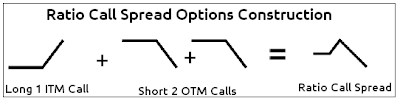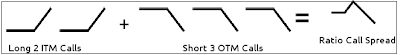Call Ratio Spread Options Payoff Functions Explained: Options, Futures, Derivatives & Commodity Trading

# Call Ratio Spread Options Payoff Functions Explained

Continuing further from our previous article on Ratio Call Spread Options Trading Example Explained, here are the details about the Payoff Functions for Ratio Call Spread Options Trading

How is a Ratio Call Spread Options Position constructed?
Ratio Call Spread Options Position is constructed using Call options (hence the name call) in a pre-determined ratio. An option trader can use 1:2 or 2:3 ratio, here in this example of Ratio Call Spread we will explain the same with a 1:2 ratio.

1) Buy 1 * ITM Call (Long)
2) Sell 2 * OTM Calls (Short)
(Want to know what is ITM, OTM, ATM in Options? See Moneyness of Options - OTM, ATM, ITM Options)

The sum of above 3 options positions give the following combination:Here is another way to construct the same payoff position for Ratio Call Spread using 2 Long calls and 3 short calls. However, please note that the more options you trade to get in and out of options, the more options trading commission you need to pay.For the remainder of this discussion, I will keep the 3 options combination for explanation of example.

Suppose the Apple stock is trading around \$50 and you don’t expect it to move much in the next 2 months time. Hence, you decide to take the Ratio call spread with the following call options:

1) Long 1 ITM call at strike price of \$45 at an option premium of \$6
2) Short 2 OTM calls at strike price of \$55 at an option premium of \$1 each

Hence, you pay \$6 for 1 Long position and receive \$2 for 2 short calls, hence a net debit of \$4 for this Ratio Call Spread Options Trading position.

## Payoff Function for Ratio Call Spread Options

Here is the payoff function for Ratio Call Spread Options without the prices being considered.
Please note that second \$55 short call (Turquoise color) is hidden behind the first \$55 short call (PINK colored), hence not visible.Now, lets add all of these 3 together to get the net ORANGE colored payoff function, which displays the profit and loss regions clearly. Please note that prices are still NOT considered:Finally, let's consider the price also. The option trader paid a net \$4 from these 3 options position combination. Since it is a net payout, we shift the ORANGE colored graph downwards to get the BROWN colored net payoff function with prices factored in:Let's continue onto explanation of this Ratio Call Spread Profit & Loss Calculations
 Posted by IT Correspondent See All Articles with